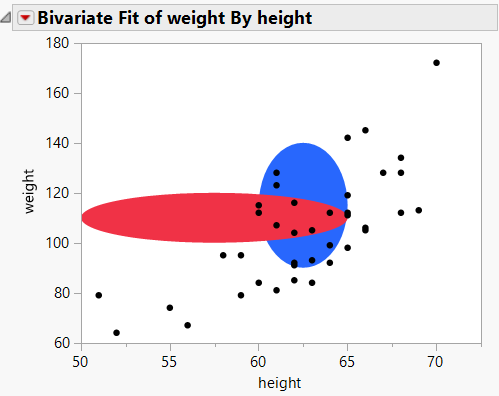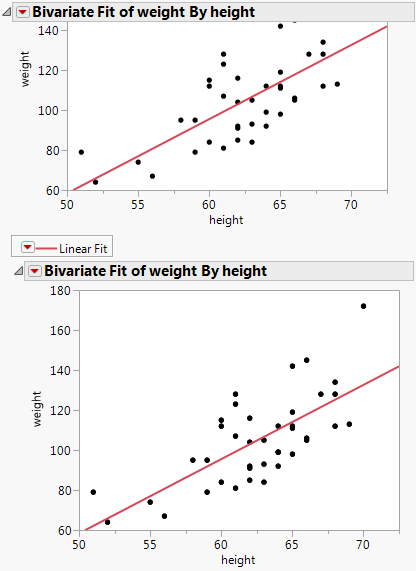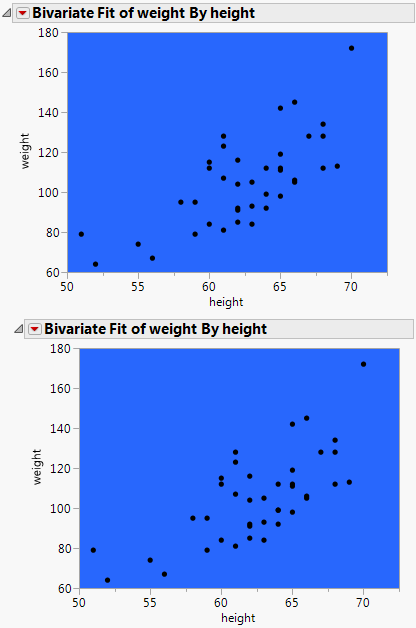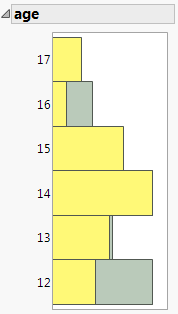Scripting Guide > Scripting Graphs > Add Scripts to Graphs > Specify the Order of Graphical Elements
Publication date: 08/13/2020

## Specify the Order of Graphical Elements

You can also add graphics elements using JSL instead of doing so interactively:

`Frame Box <<Add Graphics Script(<order>, <"description">, script)`

When you add graphical elements using JSL, they are drawn on top of whatever is already in the graph.

The optional order argument specifies in what order to draw the graphics element. The order can be the keyword Back or Front or an integer that specifies the drawing order for a number of graphics elements. For example, if you add an oval to a scatterplot, the oval is drawn on top of the markers. The keyword Back or 1 cause the oval to be drawn last. Front or 2 means the object is drawn first.

The optional Description argument is a string that appears in the Customize Graph window next to the graphics script.

To specify the drawing order for a number of graphics elements, use an integer for the order argument to determine where each is drawn in relation to the others. The following script first adds a blue oval and then adds a red oval over the blue oval. Both ovals are behind the points.

`dt = Open( "\$SAMPLE_DATA/Big Class.jmp" );`
`op = dt << Bivariate(`
`	X( :height ),`
`	Y( :weight ),`
`);`
` `
`Report( op )[Frame Box( 1 )] << Add Graphics Script(`
`	1, // draw the blue oval`
`	Description( "Blue Oval" ),`
`	Fill Color( "Blue" );`
`	Oval( 60, 140, 65, 90, 1 );`
`);`
` `
`Report( op )[Frame Box( 1 )] << Add Graphics Script(`
`	2, // draw the red oval over the blue oval`
`	Description( "Red Oval" ),`
`	Fill Color( "Red" );`
`	Oval( 50, 120, 65, 100, 1 );`
`);`

Figure 12.3 Specifying the Drawing Order### Copy and Paste Frame Contents or Settings

You can copy and paste the contents or the settings of a frame with these JSL commands:

`obj << Copy Frame Contents // contents such as a graph or line of fit`
`obj << Paste Frame Contents`
`obj << Copy Frame Settings // settings such as background color`
`obj << Paste Frame Settings`

The following example creates two Bivariate graphs, adds a line of fit to the first graph, and then copies and pastes the line to the second graph.

`dt = Open( "\$SAMPLE_DATA/Big Class.jmp" );`
`biv = dt << Bivariate( y( :weight ), x( :height ) );`
`rbiv1 = biv << Report; // create a report object`
`rbiv2 = rbiv1 << Clone Box; // create a copy of the Bivariate graph`
` `
`/* place the cloned box below the Bivariate graph`
`to create a second Bivariate graph */`
`rbiv1 << Append( rbiv2 );`
` `
`framebox1 = rbiv1[Frame Box( 1 )]; // assign references to the reports’ frame boxes`
`framebox2 = rbiv2[Frame Box( 1 )];`
`biv << Fit Line; // fit a line to the first Bivariate graph`
` `
`// copy the frame contents from framebox1`
`framebox1 << Copy Frame Contents;`
` `
`// paste the frame contents to framebox2`
`framebox2 << Paste Frame Contents;`

Figure 12.4 Copying and Pasting Frame ContentsThe following example creates two Bivariate graphs, sets the background of the first graph to blue, and then copies and pastes the blue background setting to the second graph:

`dt = Open( "\$SAMPLE_DATA/Big Class.jmp" );`
` `
`biv1 = dt << Bivariate( y( :weight ), x( :height ) );`
` `
`// create the first Bivariate graph`
`rbiv1 = biv1 << Report; // create a report object`
`rbiv2 = rbiv1 << Clone Box; // create a copy of the Bivariate graph`
` `

/* place the cloned box below the Bivariate graph

to create a second Bivariate graph */

`rbiv1 << Append( rbiv2 );`
` `
`framebox1 = rbiv1[Frame Box( 1 )]; /* assign a reference to the`
`													reports’ frame boxes */`
`framebox2 = rbiv2[Frame Box( 1 )];`
` `

// assign the background color to framebox1

`framebox1 << Background Color( "Blue" );`
` `

// copy the frame settings from framebox1

`framebox1 << Copy Frame Settings;`
` `

// paste the frame settings to framebox2

`framebox2 << Paste Frame Settings;`

Figure 12.5 Copying and Pasting the Background#### Copy and Paste Histograms

The following example shows how to create two histograms and copy the second histogram into the first histogram. A platform will not save a pasted-in segment, so you'll need to run a script like this to reproduce the graph.

`dt = Open( "\$SAMPLE_DATA/Big Class.jmp" );`
`dist = dt << Distribution(`
`	SendToByGroup( {:sex == "F"} ),`
`	Nominal Distribution( Column( :age ) ),`
`	Histograms Only,`
`	By( :sex ),`
`	SendToByGroup(`
`		{:sex == "M"},`
`		SendToReport(`
`			Dispatch(`
`				{"Distributions sex=M", "age"},`
`				"Distrib Nom Hist",`
`				FrameBox,`
`				{DispatchSeg(`
`					Hist Seg( 1 ),`
` `

// set the histogram color for males

`					Fill Color( "Light Yellow" ),`
`				)}`
`			)`
`		)`
`	)`
`);`
` `
`For( i = 2, i <= N Items( dist ), i++,`
`	Report( dist[i] )[FrameBox( 1 )] << Copy Frame Contents;`
` `

// copy the the second histogram into the first histogram

`	Report( dist )[FrameBox( 1 )] << Paste Frame Contents;`
`);`
` `
`New Window( "Distribution", Outline Box( "age", Report( dist )[Picture Box( 1 )] ) );`

Figure 12.6 Pasted Histogram#### Interactively Copy and Paste Frame Contents and Settings

To interactively copy and paste the frame settings, follow these steps:

1. Right-click the graph and select Edit > Copy Frame Settings.

2. Right-click the frame to which you want to paste the settings and select Edit > Paste Frame Settings.

To interactively copy and paste the frame contents, follow these steps:

1. Right-click the graph and select Edit > Copy Frame Contents.

2. Right-click the frame to which you want to paste the contents and select Edit > Paste Frame Contents.